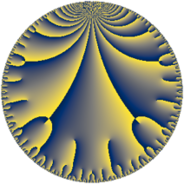# Properties

 Label 162.11.dLevel $162$ Weight $11$ Character orbit 162.d Rep. character $\chi_{162}(53,\cdot)$ Character field $\Q(\zeta_{6})$ Dimension $80$ Newform subspaces $7$ Sturm bound $297$ Trace bound $7$

# Related objects

## Defining parameters

 Level: $$N$$ $$=$$ $$162 = 2 \cdot 3^{4}$$ Weight: $$k$$ $$=$$ $$11$$ Character orbit: $$[\chi]$$ $$=$$ 162.d (of order $$6$$ and degree $$2$$) Character conductor: $$\operatorname{cond}(\chi)$$ $$=$$ $$9$$ Character field: $$\Q(\zeta_{6})$$ Newform subspaces: $$7$$ Sturm bound: $$297$$ Trace bound: $$7$$ Distinguishing $$T_p$$: $$5$$

## Dimensions

The following table gives the dimensions of various subspaces of $$M_{11}(162, [\chi])$$.

Total New Old
Modular forms 564 80 484
Cusp forms 516 80 436
Eisenstein series 48 0 48

## Trace form

 $$80 q + 20480 q^{4} - 61190 q^{7} + O(q^{10})$$ $$80 q + 20480 q^{4} - 61190 q^{7} + 1402750 q^{13} - 10485760 q^{16} + 7101676 q^{19} - 6946944 q^{22} + 97967612 q^{25} - 62658560 q^{28} + 96926740 q^{31} - 61492992 q^{34} + 560825572 q^{37} + 219665020 q^{43} + 733827840 q^{46} - 824632710 q^{49} - 718208000 q^{52} - 1882056096 q^{55} - 1247579520 q^{58} + 1318973998 q^{61} - 10737418240 q^{64} + 2205584170 q^{67} - 6242405760 q^{70} + 3640794964 q^{73} + 1818029056 q^{76} + 10320341650 q^{79} + 5925737088 q^{82} - 7038644544 q^{85} + 3556835328 q^{88} + 7044566756 q^{91} - 12832256640 q^{94} - 7644335546 q^{97} + O(q^{100})$$

## Decomposition of $$S_{11}^{\mathrm{new}}(162, [\chi])$$ into newform subspaces

Label Dim $A$ Field CM Traces $q$-expansion
$a_{2}$ $a_{3}$ $a_{5}$ $a_{7}$
162.11.d.a $4$ $102.928$ $$\Q(\sqrt{-2}, \sqrt{-3})$$ None $$0$$ $$0$$ $$0$$ $$-41272$$ $$q+(2^{4}\beta _{1}-2^{4}\beta _{3})q^{2}+(2^{9}-2^{9}\beta _{2})q^{4}+\cdots$$
162.11.d.b $4$ $102.928$ $$\Q(\sqrt{-2}, \sqrt{-3})$$ None $$0$$ $$0$$ $$0$$ $$6230$$ $$q+(8\beta _{1}-8\beta _{3})q^{2}+(2^{9}-2^{9}\beta _{2})q^{4}+\cdots$$
162.11.d.c $8$ $102.928$ $$\mathbb{Q}[x]/(x^{8} - \cdots)$$ None $$0$$ $$0$$ $$0$$ $$-11516$$ $$q-\beta _{3}q^{2}-2^{9}\beta _{1}q^{4}+(7^{2}\beta _{3}-\beta _{4}-7^{2}\beta _{6}+\cdots)q^{5}+\cdots$$
162.11.d.d $8$ $102.928$ 8.0.$$\cdots$$.97 None $$0$$ $$0$$ $$0$$ $$45112$$ $$q-\beta _{3}q^{2}-2^{9}\beta _{1}q^{4}+(26\beta _{3}+\beta _{4}+26\beta _{5}+\cdots)q^{5}+\cdots$$
162.11.d.e $16$ $102.928$ $$\mathbb{Q}[x]/(x^{16} - \cdots)$$ None $$0$$ $$0$$ $$0$$ $$-45112$$ $$q-2^{4}\beta _{8}q^{2}+(2^{9}+2^{9}\beta _{1})q^{4}+(686\beta _{8}+\cdots)q^{5}+\cdots$$
162.11.d.f $16$ $102.928$ $$\mathbb{Q}[x]/(x^{16} - \cdots)$$ None $$0$$ $$0$$ $$0$$ $$-10792$$ $$q-\beta _{4}q^{2}+(2^{9}+2^{9}\beta _{1})q^{4}+(13\beta _{2}+\beta _{10}+\cdots)q^{5}+\cdots$$
162.11.d.g $24$ $102.928$ None $$0$$ $$0$$ $$0$$ $$-3840$$

## Decomposition of $$S_{11}^{\mathrm{old}}(162, [\chi])$$ into lower level spaces

$$S_{11}^{\mathrm{old}}(162, [\chi]) \cong$$ $$S_{11}^{\mathrm{new}}(9, [\chi])$$$$^{\oplus 6}$$$$\oplus$$$$S_{11}^{\mathrm{new}}(18, [\chi])$$$$^{\oplus 3}$$$$\oplus$$$$S_{11}^{\mathrm{new}}(27, [\chi])$$$$^{\oplus 4}$$$$\oplus$$$$S_{11}^{\mathrm{new}}(54, [\chi])$$$$^{\oplus 2}$$$$\oplus$$$$S_{11}^{\mathrm{new}}(81, [\chi])$$$$^{\oplus 2}$$